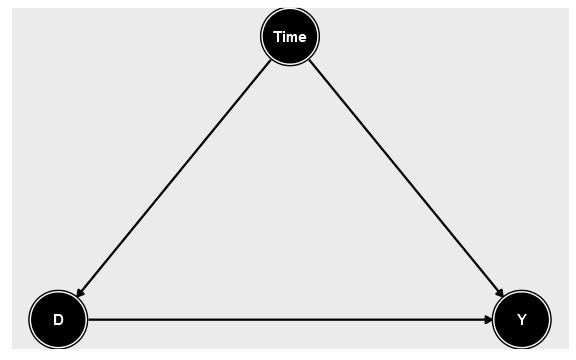# Lecture 21 Difference in Differences

## Recap

• Last time we discussed the concept of identifying a causal effect by selecting a comparable untreated group (aka “control group”) that is the same EXCEPT for the treatment, so any differences are because of treatment
• We picked a control group explicitly by matching on a set of variables
• Of course, this is the same as controlling for those variables - if the variables we match on block all back door paths, we’re good. But if not?

## Today

• Today we’re going to look at one of the most commonly used methods in causal inference, called Difference-in-Differences
• The basic idea is to sort of combine untreated groups with fixed effects
• We have a treated group that we observe both before and after they’re treated
• And we have an untreated group
• The treated and control groups probably aren’t identical - there are back doors! So… we control for group like with fixed effects

## The Basic Problem

• What kind of setup lends itself to being studied with difference-in-differences?
• Crucially, we need to have a group (or groups) that receives a treatment
• And, we need to observe them both before and after they get their treatment
• Observing each individual (or group) multiple times, kind of like we did with fixed effects

## The Basic Problem

• So one obvious thing we might do would be to just use fixed effects
• Using variation within group, comparing the time before the policy to the time after
• But!

## The Basic Problem

• Unlike with fixed effects, the relationship between time and treatment is very clear: early = no treatment. Late = treatment
• So if anything else is changing over time, we have a back door!## The Basic Problem

• Ok, time is a back door, no problem. We can observe and measure time. So we’ll just control for it and close the back door!
• But we can’t!
• Why not?
• Because in this group, you’re either before treatment and `D = 0`, or after treatment and `D = 1`. If we control for time, we’re effectively controlling for treatment
• “What’s the effect of treatment, controlling for treatment” doesn’t make any sense

## The Basic Problem

``````#Create our data
diddata <- tibble(year = sample(2002:2010,10000,replace=T)) %>%
mutate(D = year >= 2007) %>% mutate(Y = 2*D + .5*year + rnorm(10000))
#Now, control for year
diddata <- diddata %>% group_by(year) %>% mutate(D.r = D - mean(D), Y.r = Y - mean(Y))
#What's the difference with and without treatment?
diddata %>% group_by(D) %>% summarize(Y=mean(Y))``````
``````## # A tibble: 2 x 2
##   D         Y
##   <lgl> <dbl>
## 1 FALSE 1002.
## 2 TRUE  1006.``````
``````#And controlling for time?
diddata %>% group_by(D.r) %>% summarize(Y=mean(Y.r))``````
``````## # A tibble: 1 x 2
##     D.r        Y
##   <dbl>    <dbl>
## 1     0 1.84e-15``````

## The Difference-in-differences Solution

• We can add a control group that did not get the treatment
• Then, any changes that are the result of time should show up for that control group, and we can get rid of them!
• The change for the treatment from from before to after is because of both treatment and time. If we measure the time effect using our control, and subtract that out, we’re left with just the effect of treatment!

## Once Again

``````#Create our data
diddata <- tibble(year = sample(2002:2010,10000,replace=T),
group = sample(c('TreatedGroup','UntreatedGroup'),10000,replace=T)) %>%
mutate(after = (year >= 2007)) %>%
#Only let the treatment be applied to the treated group
mutate(D = after*(group=='TreatedGroup')) %>%
mutate(Y = 2*D + .5*year + rnorm(10000))
#Now, get before-after differences for both groups
means <- diddata %>% group_by(group,after) %>% summarize(Y=mean(Y))

#Before-after difference for untreated, has time effect only
bef.aft.untreated <- filter(means,group=='UntreatedGroup',after==1)\$Y - filter(means,group=='UntreatedGroup',after==0)\$Y
#Before-after for treated, has time and treatment effect
bef.aft.treated <- filter(means,group``````